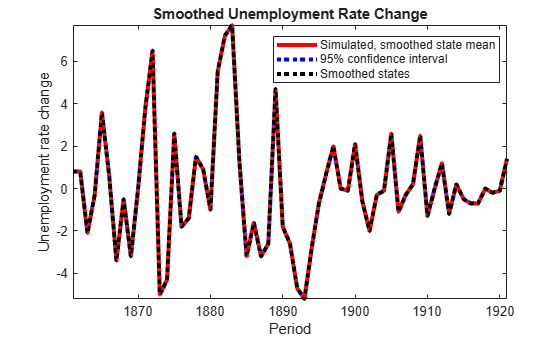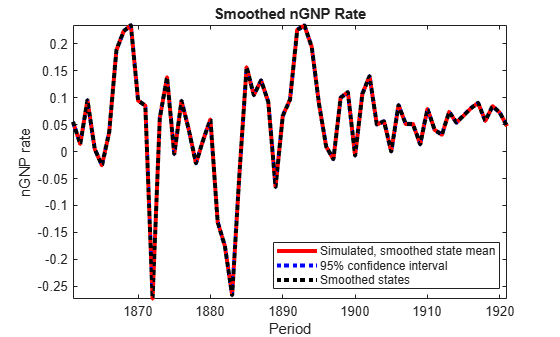# Compare Simulation Smoother to Smoothed States

This example shows how the results of the state-space model simulation smoother (`simsmooth`) compare to the smoothed states (`smooth`).

Suppose that the relationship between the change in the unemployment rate (${x}_{1,t}$) and the nominal gross national product (nGNP) growth rate (${x}_{3,t}$) can be expressed in the following, state-space model form.

`$\left[\begin{array}{c}{x}_{1,t}\\ {x}_{2,t}\\ {x}_{3,t}\\ {x}_{4,t}\end{array}\right]=\left[\begin{array}{cccc}{\varphi }_{1}& {\theta }_{1}& {\gamma }_{1}& 0\\ 0& 0& 0& 0\\ {\gamma }_{2}& 0& {\varphi }_{2}& {\theta }_{2}\\ 0& 0& 0& 0\end{array}\right]\left[\begin{array}{c}{x}_{1,t-1}\\ {x}_{2,t-1}\\ {x}_{3,t-1}\\ {x}_{4,t-1}\end{array}\right]+\left[\begin{array}{cc}1& 0\\ 1& 0\\ 0& 1\\ 0& 1\end{array}\right]\left[\begin{array}{c}{u}_{1,t}\\ {u}_{2,t}\end{array}\right]$`

`$\left[\begin{array}{c}{y}_{1,t}\\ {y}_{2,t}\end{array}\right]=\left[\begin{array}{cccc}1& 0& 0& 0\\ 0& 0& 1& 0\end{array}\right]\left[\begin{array}{c}{x}_{1,t}\\ {x}_{2,t}\\ {x}_{3,t}\\ {x}_{4,t}\end{array}\right]+\left[\begin{array}{cc}{\sigma }_{1}& 0\\ 0& {\sigma }_{2}\end{array}\right]\left[\begin{array}{c}{\epsilon }_{1,t}\\ {\epsilon }_{2,t}\end{array}\right],$`

where:

• ${x}_{1,t}$ is the change in the unemployment rate at time t.

• ${x}_{2,t}$ is a dummy state for the MA(1) effect on ${x}_{1,t}$.

• ${x}_{3,t}$ is the nGNP growth rate at time t.

• ${x}_{4,t}$ is a dummy state for the MA(1) effect on ${x}_{3,t}$.

• ${y}_{1,t}$ is the observed change in the unemployment rate.

• ${y}_{2,t}$ is the observed nGNP growth rate.

• ${u}_{1,t}$ and ${u}_{2,t}$ are Gaussian series of state disturbances having mean 0 and standard deviation 1.

• ${\epsilon }_{1,t}$ is the Gaussian series of observation innovations having mean 0 and standard deviation ${\sigma }_{1}$.

• ${\epsilon }_{2,t}$ is the Gaussian series of observation innovations having mean 0 and standard deviation ${\sigma }_{2}$.

Load the Nelson-Plosser data set, which contains the unemployment rate and nGNP series, among other things.

`load Data_NelsonPlosser`

Preprocess the data by taking the natural logarithm of the nGNP series, and the first difference of each. Also, remove the starting `NaN` values from each series.

```isNaN = any(ismissing(DataTable),2); % Flag periods containing NaNs gnpn = DataTable.GNPN(~isNaN); u = DataTable.UR(~isNaN); T = size(gnpn,1); % Sample size y = zeros(T-1,2); % Preallocate y(:,1) = diff(u); y(:,2) = diff(log(gnpn));```

This example proceeds using series without `NaN` values. However, using the Kalman filter framework, the software can accommodate series containing missing values.

Specify the coefficient matrices.

```A = [NaN NaN NaN 0; 0 0 0 0; NaN 0 NaN NaN; 0 0 0 0]; B = [1 0;1 0 ; 0 1; 0 1]; C = [1 0 0 0; 0 0 1 0]; D = [NaN 0; 0 NaN];```

Specify the state-space model using `ssm`. Verify that the model specification is consistent with the state-space model.

`Mdl = ssm(A,B,C,D)`
```Mdl = State-space model type: ssm State vector length: 4 Observation vector length: 2 State disturbance vector length: 2 Observation innovation vector length: 2 Sample size supported by model: Unlimited Unknown parameters for estimation: 8 State variables: x1, x2,... State disturbances: u1, u2,... Observation series: y1, y2,... Observation innovations: e1, e2,... Unknown parameters: c1, c2,... State equations: x1(t) = (c1)x1(t-1) + (c3)x2(t-1) + (c4)x3(t-1) + u1(t) x2(t) = u1(t) x3(t) = (c2)x1(t-1) + (c5)x3(t-1) + (c6)x4(t-1) + u2(t) x4(t) = u2(t) Observation equations: y1(t) = x1(t) + (c7)e1(t) y2(t) = x3(t) + (c8)e2(t) Initial state distribution: Initial state means are not specified. Initial state covariance matrix is not specified. State types are not specified. ```

Estimate the model parameters, and use a random set of initial parameter values for optimization. Restrict the estimate of ${\sigma }_{1}$ and ${\sigma }_{2}$ to all positive, real numbers using the `'lb'` name-value pair argument. For numerical stability, specify the Hessian when the software computes the parameter covariance matrix, using the `'CovMethod'` name-value pair argument.

```rng(1); params0 = rand(8,1); [EstMdl,estParams] = estimate(Mdl,y,params0,... 'lb',[-Inf -Inf -Inf -Inf -Inf -Inf 0 0],'CovMethod','hessian');```
```Method: Maximum likelihood (fmincon) Sample size: 61 Logarithmic likelihood: -199.397 Akaike info criterion: 414.793 Bayesian info criterion: 431.68 | Coeff Std Err t Stat Prob ---------------------------------------------------- c(1) | 0.03387 0.15213 0.22262 0.82383 c(2) | -0.01258 0.05749 -0.21876 0.82684 c(3) | 2.49856 0.22759 10.97827 0 c(4) | 0.77438 2.58648 0.29940 0.76464 c(5) | 0.13994 2.64363 0.05294 0.95778 c(6) | 0.00367 2.45477 0.00149 0.99881 c(7) | 0.00239 2.11325 0.00113 0.99910 c(8) | 0.00014 0.12685 0.00113 0.99910 | | Final State Std Dev t Stat Prob x(1) | 1.40000 0.00239 585.18164 0 x(2) | 0.21778 0.91641 0.23765 0.81216 x(3) | 0.04730 0.00014 329.58394 0 x(4) | 0.03568 0.00015 240.95607 0 ```

`EstMdl` is an `ssm` model, and you can access its properties using dot notation.

Simulate `1e4` paths of observations from the fitted, state-space model `EstMdl` using the simulation smoother. Specify to simulate observations for each period.

```numPaths = 1e4; SimX = simsmooth(EstMdl,y,'NumPaths',numPaths);```

`SimX` is a `T - 1`-by- `4`-by- `numPaths` matrix containing the simulated states. The rows of `SimX` correspond to periods, the columns correspond to a state in the model, and the pages correspond to paths.

Estimate the smoothed state means, standard deviations, and 95% confidence intervals.

```SmoothBar = mean(SimX,3); SmoothSTD = std(SimX,0,3); SmoothCIL = SmoothBar - 1.96*SmoothSTD; SmoothCIU = SmoothBar + 1.96*SmoothSTD;```

Estimate smooth states using `smooth`.

`SmoothX = smooth(EstMdl,y);`

Plot the smoothed states, and the means of the simulated states and their 95% confidence intervals.

```figure h = plot(dates(2:T),SmoothBar(:,1),'-r',... dates(2:T),SmoothCIL(:,1),':b',... dates(2:T),SmoothCIU(:,1),':b',... dates(2:T),SmoothX(:,1),':k',... 'LineWidth',3); xlabel 'Period'; ylabel 'Unemployment rate'; legend(h([1,2,4]),{'Simulated, smoothed state mean','95% confidence interval',... 'Smoothed states'},'Location','Best'); title 'Smoothed Unemployment Rate'; axis tight``````figure h = plot(dates(2:T),SmoothBar(:,3),'-r',... dates(2:T),SmoothCIL(:,3),':b',... dates(2:T),SmoothCIU(:,3),':b',... dates(2:T),SmoothX(:,3),':k',... 'LineWidth',3); xlabel 'Period'; ylabel 'nGNP'; legend(h([1,2,4]),{'Simulated, smoothed state mean','95% confidence interval',... 'Smoothed states'},'Location','Best'); title 'Smoothed nGNP'; axis tight```The simulated state means are practically identical to the smoothed states.